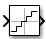# Quantizer

Discretize input at given interval

•Libraries:

## Description

The Quantizer block discretizes the input signal using a quantization algorithm. The block uses a round-to-nearest method to map signal values to quantized values at the output that are defined by the Quantization interval. A smooth input signal can take on a stair-step shape after quantization.

This equation mathematically describes the round-to-nearest method:

`y = q * round(u/q) `

where `y` is the quantized output, `u` is the input, and `q` is the Quantization interval.

## Ports

### Input

expand all

Input signal to the quantization algorithm.

Data Types: `single` | `double`
Complex Number Support: Yes

### Output

expand all

Output signal composed of discrete values, quantized with a round-to-nearest approach:

`y = q * round(u/q) `

where `y` is the output, `u` is the input, and `q` is the Quantization interval.

Data Types: `single` | `double`

## Parameters

expand all

Specify the quantization interval used in the algorithm. Quantization levels for the Quantizer block output are integer multiples of the Quantization interval.

#### Programmatic Use

 Block Parameter: `QuantizationInterval` Type: character vector Value: Any real or complex value Default: `'0.5'`

The linearization commands in Simulink® software treat this block as a gain in state space. Select this check box to cause the commands to treat the gain as 1. Clear the box to have the commands treat the gain as 0.

#### Programmatic Use

 Block Parameter: `LinearizeAsGain` Type: character vector Value: `'off'` | `'on'` Default: `'on'`

Specify the time interval between samples. To inherit the sample time, set this parameter to `-1`. For more information, see Specify Sample Time.

#### Dependencies

This parameter is visible only if you set it to a value other than `-1`. To learn more, see Blocks for Which Sample Time Is Not Recommended.

#### Programmatic Use

 Block Parameter: `SampleTime` Type: string scalar or character vector Default: `"-1"`

## Block Characteristics

 Data Types `double` | `single` Direct Feedthrough `yes` Multidimensional Signals `no` Variable-Size Signals `no` Zero-Crossing Detection `no`

## Version History

Introduced before R2006a